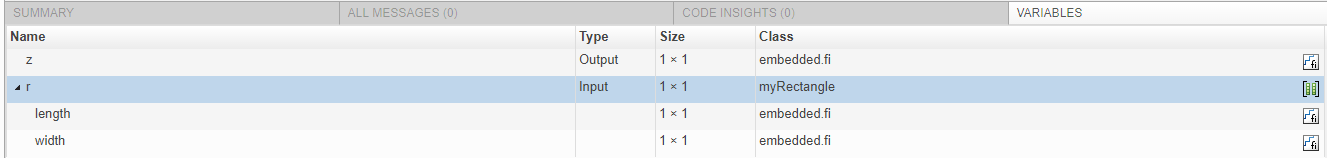Documentation

## Specify Objects as Inputs

When you accelerate code by using `fiaccel`, to specify the type of an input that is a value class object, you can provide an example object with the `-args` option.

1. Define the value class. For example, define a class `myRectangle`.

```classdef myRectangle properties length; width; end methods function obj = myRectangle(l,w) if nargin > 0 obj.length = l; obj.width = w; end end function area = calcarea(obj) area = obj.length * obj.width; end end end```

2. Define a function that takes an object of the value class as an input. For example:

```function z = getarea(r) %#codegen z = calcarea(r); end```

3. Define an object of the class.

```rect_obj = myRectangle(fi(4),fi(5)) ```

```rect_obj = myRectangle with properties: length: [1×1 embedded.fi] width: [1×1 embedded.fi]```
4. Pass the example object to `fiaccel` by using the `-args` option.

`fiaccel getarea -args {rect_obj} -report`

In the report, you see that `r` has the same properties, `length` and `width`, as the example object `rect_object`.Instead of providing an example object, you can create a type for an object of the value class and provide the type with the `-args` option.

1. Define an object of the class:

`rect_obj = myRectangle(fi(4),fi(5))`
```rect_obj = myRectangle with properties: length: [1×1 embedded.fi] width: [1×1 embedded.fi]```

2. To create a type for an object of `myRectangle` that has the same property types as `rect_obj`, use `coder.typeof`. `coder.typeof` creates a `coder.ClassType` object that defines a type for a class.

```t= coder.typeof(rect_obj) ```
```t = coder.ClassType 1×1 myRectangle length: 1×1 embedded.fi DataTypeMode: Fixed-point: binary point scaling Signedness: Signed WordLength: 16 FractionLength: 12 width : 1×1 embedded.fi DataTypeMode: Fixed-point: binary point scaling Signedness: Signed WordLength: 16 FractionLength: 12```

3. Pass the type to `fiaccel` by using the `-args` option.

`fiaccel getarea -args {t} -report`

After you create the type, you can change the types of the properties.

```t.Properties.length = coder.typeof(fi(0,1,32,29)) t.Properties.width = coder.typeof(fi(0,1,32,29))```

You can also add or delete properties. For example, to add a property `newprop`:

```t.Properties.newprop = coder.typeof(int16(1)) ```

### Consistency Between `coder.ClassType` Object and Class Definition File

When you accelerate code, the properties of the `coder.ClassType` object that you pass to `fiaccel` must be consistent with the properties in the class definition file. If the class definition file has properties that your code does not use, the `coder.ClassType` object does not have to include those properties. `fiaccel` removes properties that you do not use.

### Limitations for Using Objects as Entry-Point Function Inputs

Entry-point function inputs that are objects have these limitations:

• An object that is an entry-point function input must be an object of a value class. Objects of handle classes cannot be entry-point function inputs. Therefore, a value class that contains a handle class cannot be an entry-point function input.

• An object cannot be a global variable.

• If an object has duplicate property names, you cannot use it with `coder.Constant`. Duplicate property names occur in an object of a subclass in these situations:

• The subclass has a property with the same name as a property of the superclass.

• The subclass derives from multiple superclasses that use the same name for a property.

For information about when MATLAB® allows duplicate property names, see Subclassing Multiple Classes (MATLAB).